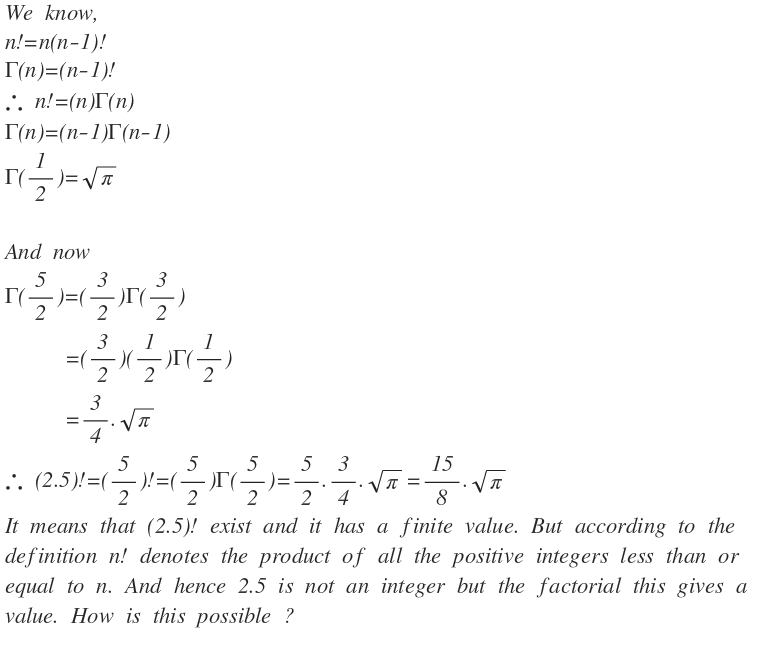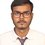# Where is the fallacy?Note by Trishit Chandra
6 years, 5 months ago

This discussion board is a place to discuss our Daily Challenges and the math and science related to those challenges. Explanations are more than just a solution — they should explain the steps and thinking strategies that you used to obtain the solution. Comments should further the discussion of math and science.

When posting on Brilliant:

• Use the emojis to react to an explanation, whether you're congratulating a job well done , or just really confused .
• Ask specific questions about the challenge or the steps in somebody's explanation. Well-posed questions can add a lot to the discussion, but posting "I don't understand!" doesn't help anyone.
• Try to contribute something new to the discussion, whether it is an extension, generalization or other idea related to the challenge.

MarkdownAppears as
*italics* or _italics_ italics
**bold** or __bold__ bold
- bulleted- list
• bulleted
• list
1. numbered2. list
1. numbered
2. list
Note: you must add a full line of space before and after lists for them to show up correctly
paragraph 1paragraph 2

paragraph 1

paragraph 2

[example link](https://brilliant.org)example link
> This is a quote
This is a quote
    # I indented these lines
# 4 spaces, and now they show
# up as a code block.

print "hello world"
# I indented these lines
# 4 spaces, and now they show
# up as a code block.

print "hello world"
MathAppears as
Remember to wrap math in $$ ... $$ or $ ... $ to ensure proper formatting.
2 \times 3 $2 \times 3$
2^{34} $2^{34}$
a_{i-1} $a_{i-1}$
\frac{2}{3} $\frac{2}{3}$
\sqrt{2} $\sqrt{2}$
\sum_{i=1}^3 $\sum_{i=1}^3$
\sin \theta $\sin \theta$
\boxed{123} $\boxed{123}$

Sort by:

There's another definition for the gamma function. Check it out here: Gamma Function

- 6 years, 5 months ago

I saw that there is another definition of gamma function but there is not another definition of factorial which implies the above derivation. And as the formulas I have written in the first portion are correct then the derivation should be correct but there is a contradiction between the definition of factorial and the formulas.

- 6 years, 5 months ago

Definitions can be extended to include more cases than what you initially dealt with.

A typical example that you should be familiar with, is that in (say) 2nd grade you were told that "multiplication is just repeated addition. To find $4 \times 5$, you draw out 4 groups of 5 items, and count them to get 20". However, that "logic" quickly fails when you try and extend it to frations / irrational numbers. Pray tell, how do you draw out $\sqrt{2}$ groups of $\pi$ items and count them?

The next example would be exponentiation, which is (similarly) often introduced as "exponentiation is just repeated multiplication" To find $4 ^ 5$, we do $4 \times 4 \times 4 \times 4 \times 4$. Pray tell, how would you write $\sqrt{2} ^ \pi$?

Staff - 6 years, 5 months ago

So, is the process of this derivation wrong ?

- 6 years, 5 months ago

So you are saying that the factorial of fraction should be possible but this can not be possible according to the definition of it. And hence the derivation is correct.

- 6 years, 5 months ago

We can take the factorial of fractions, and or irrational numbers. The definition of it is not $x ! = x \times (x-1) \times (x-2) \times \ldots$ where the last term is between 0 and 1.

We can also extend this to negative numbers, but not to the negative integers.

The above derivation is correct, but it does not explain how it calculated $\Gamma ( \frac{1}{2} )$. With that as the working assumption (ie not proven), the rest of the steps look correct.

Staff - 6 years, 5 months ago

Okay. Thank you Calvin Lin for helping me. Now I've understood. Can you refer me some link or book where I can find this conception of factorial because Wikipedia is saying that factorial means the product of the positive integers less the or equal to the number. And you said in the last line bracket that something is not proven, what is that?

- 6 years, 5 months ago

See Trishit, a special form of the gamma function (the one you have mentioned in your problem) is actually an improper integral of the following form: $\Gamma (t)=\int _{ 0 }^{ \infty }{ { x }^{ t-1 }{ e }^{ -x }dt }$ the domain of this function being the set of all complex numbers t, with Re(t)>=0. The gamma function reduces to the simple factorial function defined by $n!=\prod _{ i=1 }^{ n }{ i } ,when\quad n\ge 1$ and $n!=1,when\quad n=0$ which has its domain the set of non-negative integers. Factorial function turns out to be a very special form of the gamma function in the set of non-negative integers. Clearly, the domain of the factorial function does not contain fractions like 2.5 and thus (2.5)! is not defined. Your fallacy is that you are considering the factorial and gamma functions to be the same. Well, you cannot call two functions the same if their domains are different.

Got it. And thank you very much.

- 6 years ago

Welcome. You can learn more about functions like gamma and beta in the topic of improper integrals There you will learn why $\Gamma \left( \frac { 1 }{ 2 } \right) =\sqrt { \pi }$. This result can be proved from the Euler's reflection formula. I give a short sketch of the proof here. The reflection formula states that(this can also be proved):$\Gamma (t)\Gamma (1-t)=\frac { \pi }{ sin\quad \pi t } \\ \Longrightarrow \Gamma \left( \frac { 1 }{ 2 } \right) \Gamma \left( 1-\frac { 1 }{ 2 } \right) =\Gamma \left( \frac { 1 }{ 2 } \right) \Gamma \left( \frac { 1 }{ 2 } \right) ={ \left\{ \Gamma \left( \frac { 1 }{ 2 } \right) \right\} }^{ 2 }=\frac { \pi }{ sin\quad \frac { \pi }{ 2 } } =\pi$$\Longrightarrow \Gamma \left( \frac { 1 }{ 2 } \right) =\sqrt { \pi }$

Thanks for providing the insight!

Could you look over this Gamma Function wiki page and provide us with feedback about areas that it can be improved?

Staff - 6 years ago